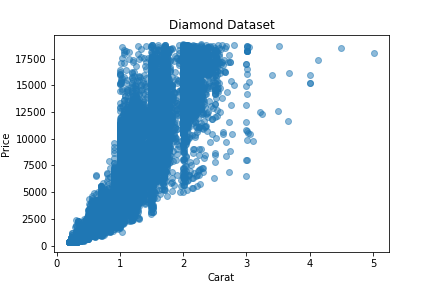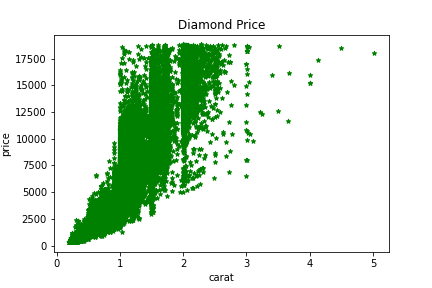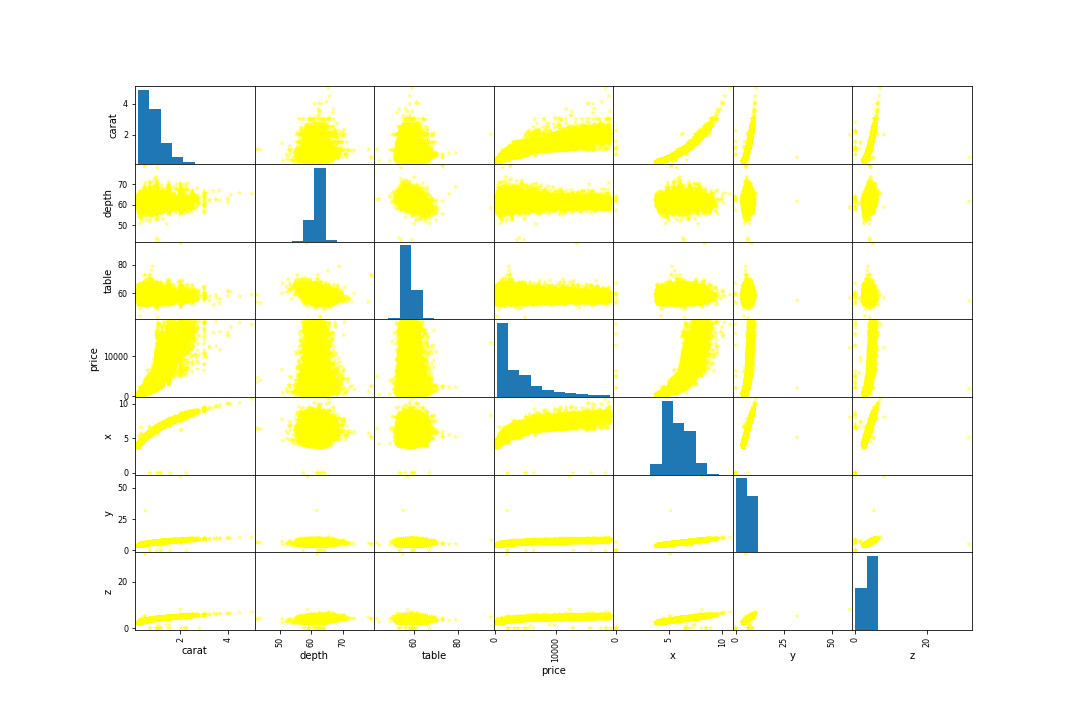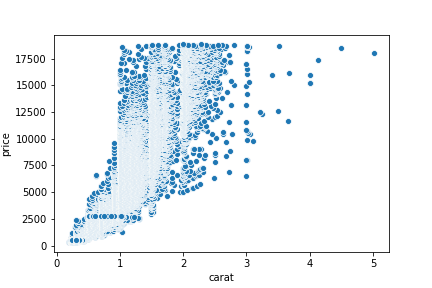# How to create Scatter Plot in Python

## Introduction –

Data Visualization is necessary and indeed a very interesting scope of work while solving any Data Science problem. There are several licensed and open-source Data Visualization tools available in the market like Tableau, Power BI, DataWrapper, Infogram, etc. Having said that, Python is in no way behind and provides some amazing libraries to perform Data Visualization activities. In relation to Python Programming Language, we have established some fundamental concepts in our previous few tutorials like Python Data Types, Loops in Python. There are various ways to visualize data by creating Histogram, Bar Plot, Scatter Plot, Box Plot, Heat Map, Line Chart, etc. In this article, we are going to look at how to create a scatter plot in Python using the widely used libraries like Pandas, Seaborn, Matplotlib, etc.

The data set we are going to use for our charts is the Diamond data from the Kaggle website. Let us import the diamonds.csv and create a data frame out of it in Python using Pandas. We can see the first few rows of the data frame as well using the head command.

```import pandas as pd
import seaborn as sns
!pip install matplotlib
import matplotlib.pyplot as plt
from pandas import ExcelWriter
from pandas import ExcelFile

%matplotlib inline

DiamondPrices = DiamondPrices.drop('Unnamed: 0', axis =1)
``

The ‘Price’ column is our target variable or the dependent variable. Other columns are the independent variables in this data set.

## Scatter Plot –

We begin our Data Visualization with Scatter Plot which can be created using Pandas, Matplotlib or even Seaborn library. Let us generate the scatter plot using the libraries one by one.

### Scatter Plot using Matplotlib –

```# create a figure and axis
fig, ax = plt.subplots()

x = DiamondPrices['price']
y = DiamondPrices['carat']

# scatter the price against the carat
ax.scatter(x,y)

# set a title and labels
ax.set_title('Diamond Dataset')
ax.set_xlabel('Price')
ax.set_ylabel('Carat')

#save the plot figure
fig.savefig('scatter_plot_matplotlib.png')```
```#display the plot
plt.show()```Can you recognize the correlation between Carat and Price?

### Scatter Plot Using Pandas –

`DiamondPrices.plot.scatter(x='carat', y='price', title='Diamond Price', marker='*', color='green').get_figure().savefig(r'Pandas Scatter Plot.png')`As is seen, it is a very simple one-line command which helps us generate the plot. We are also able to save the plot figure. Now let us see another beauty of the Pandas library in creating a scatter matrix of all the quantitative columns in the data frame. We pass the whole data frame as an argument in the scatter_matrix command. It considers only the numerical columns for plotting by default.
```pd.plotting.scatter_matrix(DiamondPrices, figsize=[15,10], marker ='*', color = 'yellow')
plt.savefig(r'Pandas Scatter Matrix Plot.png')```### Scatter Plot Using Seaborn –

```sns.scatterplot(x='carat', y='price', data=DiamondPrices)
plt.savefig('seaborn scatter plot.png')```To create a scatter matrix plot similar to what we created for all the quantitative variables in the data frame using the Pandas library, we can use the below command.

```sns.set(style="ticks")
sns.set_palette("husl")
sns.pairplot(DiamondPrices)
plt.savefig('seaborn scatter pair plot.png')```

## Conclusion –

Do read the documentation of these commands to get a clear understanding of various arguments one can pass. Like Seaborn Scatter Pair Plot documentation. I hope that you practice these scatter plot commands on your own data sets and get a grip on them. Next, we are going to create Histogram and Bar Plots using various libraries. Stay Tuned!